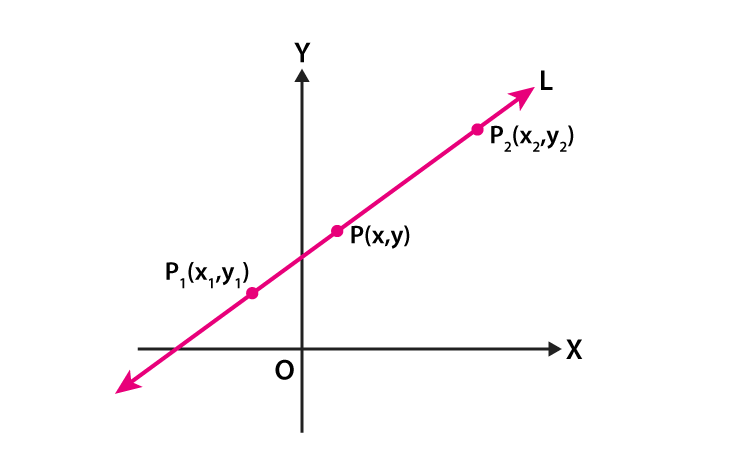Checkout JEE MAINS 2022 Question Paper Analysis : Checkout JEE MAINS 2022 Question Paper Analysis :

# Two Point Form

In geometry, a line is a set of points that is extended infinitely in two distinct ways. In other words, a line is made when we extend the two end points in either direction endlessly. Thus, we can say that a line has no end points. However, it is a one-dimensional geometric figure since it has only length but does not have width. Algebraically, we can write the different form of equations of lines based on the given information such as point slope form, slope-intercept form, two point form. In this article, you will learn how to write the equation of a line in two point form and how to derive this equation. Also, get the formula and solved examples on the two point form of line equation.

## Two Point Form Formula

The formula of two point form of a equation is given below:

Let (x1, y1) and (x2, y2) be the two points such that the equation of line passing through these two points is given by the formula:

$$\begin{array}{l}\large \frac{y-y_{1}}{x-x_{1}}=\frac{y_{2}-y_{1}}{x_{2}-x_{1}}\end{array}$$

Or

$$\begin{array}{l}\large {y-y_{1}}=\frac{y_{2}-y_{1}}{x_{2}-x_{1}}(x-x_{1})\end{array}$$

Let’s derive the two point form of a line equation.

## Two Point Form of the Equation of a Line

Let P1 (x1, y1) and P2 (x2, y2) be the two given points on the line L.

Let P(x, y) be a general point on the line L.From the figure, we can say that the three points P1, P2 and P are collinear.

That means,

Slope of P1P = Slope of P1P2

The ratio of difference of y-coordinates of P and P1 to the difference of x-coordinates of these points = The ratio of difference of y-coordinates of P1 and P2 to the difference of x-coordinates of these points

$$\begin{array}{l}\frac{y-y_{1}}{x-x_{1}}=\frac{y_{2}-y_{1}}{x_{2}-x_{1}}\end{array}$$

Or

$$\begin{array}{l}{y-y_{1}}=\frac{y_{2}-y_{1}}{x_{2}-x_{1}}(x-x_{1})\end{array}$$

Thus, equation of the line passing through the points (x1, y1) and (x2, y2) is given by

$$\begin{array}{l}{y-y_{1}}=\frac{y_{2}-y_{1}}{x_{2}-x_{1}}(x-x_{1})\end{array}$$

This is the equation of a line in two-point form.

Go through the solved examples and practice questions given below for better understanding of the concept.

### Two Point Form Examples

Example 1:

Find the equation of a line passing through the points (-2, 3) and (3, 5).

Solution:

Let the given points be:

(x1, y1) = (-2, 3)

(x2, y2) = (3, 5)

The equation of a straight line passing through the points (x1, y1) and (x2, y2) is given as:

$$\begin{array}{l}{y-y_{1}}=\frac{y_{2}-y_{1}}{x_{2}-x_{1}}(x-x_{1})\end{array}$$

Substituting the values, we get;

$$\begin{array}{l}{y-3}=\frac{5 – 3}{3-(-2)}[x -(2)]\end{array}$$

y – 3 = (2/5) (x + 2)

5(y – 3) = 2(x + 2)

5y – 15 = 2x + 4

2x + 4 – 5y + 15 = 0

2x – 5y + 19 = 0

Hence, this is the required equation of a line passing through the given points.

Example 2:

What is the equation of a straight line passing through the points (2, 0) and (0, 2)?

Solution:

Let the given points be:

(x1, y1) = (2, 0)

(x2, y2) = (0, 2)

The equation of a straight line passing through the points (x1, y1) and (x2, y2) is given as:

$$\begin{array}{l}{y-y_{1}}=\frac{y_{2}-y_{1}}{x_{2}-x_{1}}(x-x_{1})\end{array}$$

Substituting the values, we get;

$$\begin{array}{l}{y-0}=\frac{2 – 0}{0 – 2}(x – 2)\end{array}$$

y = (-2/2) (x – 2)

y = -1(x – 2)

y = -x + 2

x + y – 2 = 0

Therefore, the equation of a line passing through the given points (2, 0) and (0, 2) is x + y – 2 = 0.

### Practice Problems

1. Write the equation of the line through the points (3, –3) and (1, 5).

2. Find the equation of a straight line passing through the points (–4, 2) and (6, -7).

3. What is the equation of the line joining the points (6, 3) and (-5, 2)?

To get more knowledge on equations of a line in different forms, visit www.byjus.com and download BYJU’S – The Learning App to get interactive videos on maths and science concepts.